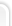## Большая коллекция рефератов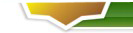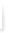### Опросы

Оцените наш сайт?
 Хорошо Отлично Полезно было посетить Добавил в закладки Еще зайду не раз Теперь я здесь постоянный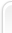# Imitating modelling of a cattle-breeding complex on basis Cedar Bog

Imitating modelling of a cattle-breeding complex on basis Cedar Bog

Setting a task of modelling. There is a cattle herd structured on the basic of age. The herd consists of Heifers, Calves, Milk cows, Cows, Bulls. Each Animal has its Age. The daily increase depends in weight of an animal which defines the weight of it depends on the age. So, for example, at achievement of the certain age (≈500 days) calves become bulls and pass from Calves in Bulls, but not all of them. Only the quantity which is enough for reproduction of the herd. The others remain calves until they fatten the necessary weight (≈1000 kg). As soon as it occurs, they are slaughtered. The similar situation is with heifers. Only at achievement of the certain age (≈450 days) they are divided into two categories: milk cows and cows. Cows are bred for meat production, MilkCows - for reproduction of herd and production of milk. According to financial opportunities a farmer defines an initial livestock of the cattle, and then makes a plan of reception of a livestock of the cattle in the future. It is required to model the change of an annual turnover of the herd and its reproduction.

Component of a high level: Farm (Farm) which describes the structure of the model.

HIGH LEVEL COMPONENT FARM_High

SUBCOMPONENTS

Meteo,

Forage,

Effluent,

Herd

COMPONENT CONNECTIONS

Meteo.sun --> Forage.sun;

Meteo.sun --> Effluent.sun;

Meteo.sun --> Herd.sun;

Herd.Dung --> Effluent.Dung;

Effluent.A --> Herd.A;

Herd.Numb_young --> Forage.Numb_young;

Herd.Numb_old --> Forage.Numb_old;

END OF FARM_High

Basic components:

1. Meteo which allows to watch the solar activity during a year (it is based on the data of the model Cedar Bog)

DYNAMIC BEHAVIOUR

suns:= 95.9[kJ/m^2] * (1+0.635 * SIN (2[1/d] * Pi *T/365));

WHENEVER T >= TNext DO

sun^:= suns - Oblako;

TNext^:= TNext + 1[d];

END

END OF Meteo

2. Forage. Norms and diets of feeding are defined in it.

DYNAMIC BEHAVIOUR

sun_bio:= sun * Bio_Fac;

WHENEVER T >= TNext DO

TNext^:= TNext +1[d];

Seno^:= 9 * Numb_young + 14 * Numb_old;

Silos^:= 8 * Numb_young + 12 * Numb_old;

Kombicorm^:= 5 * Numb_young + 7 * Numb_old;

Koncentrat^:= 2 * Numb_young + 3 * Numb_old;

Fosfat^:= 0.04 * Numb_young + 0.06 * Numb_old;

Sol^:= 0.03 * Numb_young + 0.05 * Numb_old;

END

DIFFERENTIAL EQUATIONS

p':= sun_bio - 4.03[1/a] * p;

BioMassa':= 0.48[1/a] * p - 17.87[1/a] * BioMassa;

END

END OF Forage

3. Herd, describing variable statuses and dynamics of behaviour of the herd as a whole. The important component which defines the structure of the herd, its internal interaction. It allows to watch a livestock of the herd, its reproduction.

# Changing of weight and age of heifers

WHENEVER (Stage = 1) AND (Index <= NUMBER(Heifers)) DO

Index^:= Index + 1;

Heifers: Animal[Index].Age^:= Heifers:Animal[Index].Age + 1;

Dung^:= Dung + 10;

IF Heifers:Animal[Index].Age < 180 DO

Heifers:Animal[Index].Weight^:= Heifers:Animal[Index].Weight + ves_2;

END

ELSE DO

Heifers:Animal[Index].Weight^:= Heifers:Animal[Index].Weight + ves_3;

END

END

WHENEVER (Stage = 1) AND (Index > NUMBER(Heifers)) DO

Stage^:=2;

Index^:=1;

END

# Fecundation of heifers

WHENEVER (Stage = 3) AND (Index <= NUMBER(Heifers)) DO

Index^:= Index + 1;

IF Heifers: Animal [Index].Age > (450 + Norm_raspr(10,3)) DO

IF Rand < 0.85 DO

Heifers^: TO MilkCows SEND Animal[Index]

CHANGING

Age^:=Heifers:Animal[Index].Age;

Weight^:= Heifers:Animal[Index].Weight;

Status^:= TRUE;

Stelnost^:= 1;

END

END

ELSE DO

Heifers^: TO Cows SEND Animal[Index]

CHANGING

Age^:= Heifers:Animal[Index].Age;

Weight^:= Heifers:Animal[Index].Weight;

END

END

END

END

WHENEVER (Stage = 3) AND (Index > NUMBER(Heifers)) DO

Stage^:=4;

Index^:=1;

END

# Calves became Bulls, or they are slaughtered

WHENEVER (Stage = 4) AND (Index <= NUMBER(Calves)) DO

Index^:= Index + 1;

IF (Calves:Animal[Index].Age > (500 + Norm_raspr(10,2)))

AND (NUMBER(Bulls) < 3) DO

Calves^: TO Bulls SEND Animal[Index]

CHANGING

Age^:= Calves:Animal[Index].Age;

Weight^:= Calves:Animal[Index].Weight;

END

END

IF (Calves:Animal[Index].Age > (500 + Norm_raspr(10,2)))

AND (Calves:Animal[Index].Weight > 1000) DO

VesTyw^:= VesTyw + Calves:Animal[Index].Weight/2;

Calves^: REMOVE Animal[Index];

UBOI^:= UBOI + 1;

END

END

WHENEVER (Stage = 4) AND (Index > NUMBER(Calves)) DO

Stage^:=5;

Index^:=1;

END

# Bulls are slaughtered

WHENEVER (Stage = 5) AND (Index <= NUMBER(Bulls)) DO

Index^:= Index + 1;

Bulls:Animal[Index].Age^:= Bulls:Animal[Index].Age + 1;

Dung^:= Dung + 20;

IF Bulls:Animal[Index].Weight < 1000 DO

Bulls:Animal[Index].Weight^:= Bulls:Animal[Index].Weight + ves_3;

END

IF Bulls:Animal[Index].Age > 3000 DO

VesTyw^:= VesTyw + Bulls:Animal[Index].Weight/2;

Bulls^: REMOVE Animal[Index];

UBOI^:= UBOI + 1;

END

END

WHENEVER (Stage = 5) AND (Index > NUMBER(Bulls)) DO

Stage^:=6;

Index^:=1;

END

# Birth of young animal

WHENEVER (Stage = 7) AND (Index <= NUMBER(MilkCows)) DO

Index^:= Index + 1;

MilkCows:Animal[Index].Age^:=MilkCows:Animal[Index].Age + 1;

Dung^:= Dung + 20;

IF MilkCows:Animal[Index].Weight < 600 DO

MilkCows:Animal[Index].Weight^:= MilkCows:Animal[Index].Weight + ves_3;

END

MilkCows:Animal[Index].Stelnost^:=

MilkCows:Animal[Index].Stelnost + 1;

IF MilkCows:Animal[Index].Stelnost > (275 + Norm_raspr(5,1))

AND (MilkCows:Animal[Index].Status = TRUE) DO

MilkCows:Animal[Index].Status^:= FALSE;

MilkCows:Animal[Index].Kol_voOtelov^:=

MilkCows:Animal[Index].Kol_voOtelov + 1;

IF Rand < 0.7 DO

CHANGING

Weight^:= ves_1;

Age^:= 1;

END

END

ELSE DO

CHANGING

Weight^:= ves_1;

Age^:= 1;

END

END

END

# MilkYield

IF (MilkCows:Animal[Index].Kol_voOtelov >= 1)

AND ((MilkCows:Animal[Index].Stelnost < 250) OR

(MilkCows:Animal[Index].Stelnost >280)) DO

END

# MilkCows are slaughtered

IF MilkCows:Animal[Index].Kol_voOtelov > 5 DO

VesTyw^:= VesTyw + MilkCows:Animal[Index].Weight/2;

MilkCows^: REMOVE Animal[Index];

UBOI^:= UBOI + 1;

END

END

WHENEVER (Stage = 7) AND (Index > NUMBER(MilkCows)) DO

Stage^:=8;

Index^:=1;

END

# Fecundation of MilkCows

WHENEVER (Stage = 8) AND (Index <= NUMBER(MilkCows)) DO

Index^:= Index + 1;

IF (MilkCows:Animal[Index].Status = FALSE) AND

(MilkCows:Animal[Index].Stelnost > (340)) DO

IF Rand < 0.98 DO

MilkCows:Animal[Index].Status^:= TRUE;

MilkCows:Animal[Index].Stelnost^:= 1;

END

ELSE DO

MilkCows^: TO Cows SEND Animal[Index]

CHANGING

Age^:= MilkCows:Animal[Index].Age;

Weight^:= MilkCows:Animal[Index].Weight;

END

END

END

END

END OF Herd

4. Effluent (Drains) in which it is provided annual downcast drains on fields (for fertilizer of pastures). Also sale of drains for the manufacture of mineral fertilizers is possible.

DYNAMIC BEHAVIOUR

WHENEVER T >= TNext DO

TNext^:= TNext + 1[d];

IF ((sun > 100[kJ/m^2]) AND (sun < 110[kJ/m^2])) AND (FLAG = TRUE)

AND (Dung >= 10000) DO

A^:= 0;

FLAG^:= FALSE;

END

END

WHENEVER (T >= TNext) AND (FLAG = FALSE) DO

IF (sun > 50[kJ/m^2]) AND (sun < 60[kJ/m^2]) DO

FLAG^:= TRUE;

END

END

END OF Effluent

5. Profit, expenses (profit, expenses), including an economic part of the model. Unfortunately, because of insufficient amount of the information it is designed only in the theory. But further, at the profound studying of the model, it will be created and, I hope, it will work on a level with other components of the model.

MOBILE COMPONENT Animal

DECLARATION OF ELEMENTS

STATE VARIABLES

DISCRETE

Age (INTEGER):= 0,

Weight (REAL):= 0,

Status (LOGICAL):= FALSE, # Fecundation / not Fecundation

Stelnost (INTEGER):= 0, # Duration of the pregnancy

Kol_voOtelov (INTEGER):= 0, # Number of calves

MilkYield (REAL):= 0,

Manure (REAL):= 0

END OF Animal

The results of modelling with an initial livestock of the cattle - 30 heifers, 10 calves (all animals are about one year):

On the given schedules dynamics of transition of animals from one age groups in others is displayed.

We can see, that at achievement of the certain age heifers (a red line) are divided into two categories: milk cows (a green line) and cows (a blue line). Cows fatten the necessary weight, and then they are slaughtered. Through the certain time interval Milk cows give a gain of the herd and start to be milked.

We can see, that at achievement of the certain age three calves (a red line) become bulls (a blue line), the others remain calves, until they fatten the necessary weight. As soon as it occurs, they are slaughtered.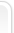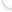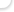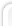Все права защищены © 2010